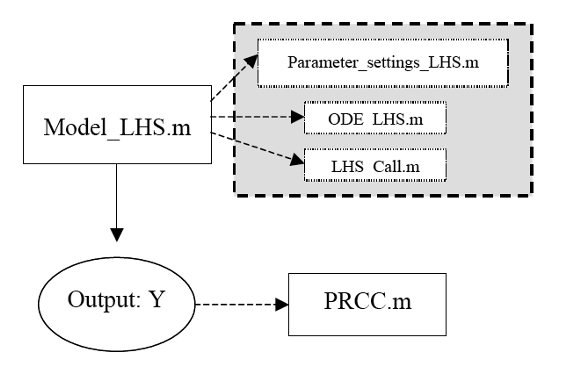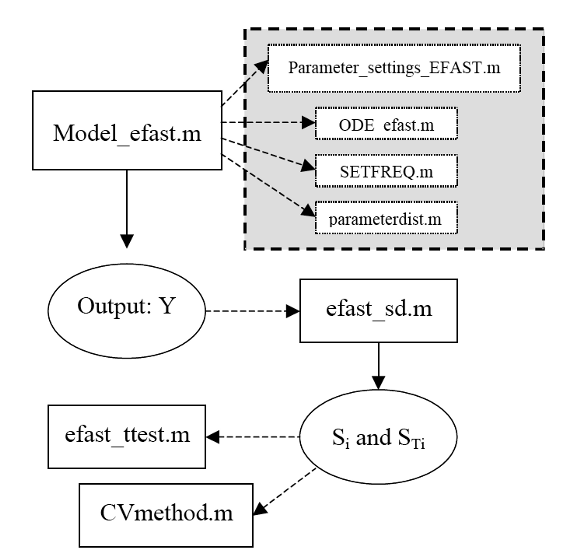[ Uncertainty And Sensitivity Analysis | Home ] All content and files © 2007, 2008 - Dr. Denise Kirschner - University of Michigan

# Uncertainty and sensitivity functions and implementation

We implemented many scripts and functions to perform uncertainty and sensitivity analysis (for PRCC and eFAST) and display scatter plots (for sample-based methods only). The scripts are written in Matlab©1 and they are available at the links below. The Statistical Toolbox is required to run them. The ODE model in section 4.2 is used as a template to illustrate the functions.

Tables 1 and 2 lists and describes all the scripts/functions implemented in Matlab for our US analysis. For the details are included at the beginning of each file. Figures 1 and 2 illustrate a diagram of how LHS-PRCC and eFAST is implemented in Matlab.Figure 1: LHS-PRCC diagram. Model_LHS calls the functions in the grey box and produces the output Y. The function PRCC needs the output Y and the LHS matrix (generated by LHS_call) to compute PRC coefficients.

LHS-PRCC

We implemented serveral different functions to perform uncertainty and sensitivity analysis and interpret the results with LHS-PRCC. The LHS-PRCC diagram (Figure 1) describes how the Matlab scripts are connected to each other and how US analysis is performed. We also have three Matlab functions to display scatter plots of LHS values versus output for the sensitivity analysis. They also calculate the correspondent sample-based correlation coefficient (CC_PLOT, RCC_PLOT and PRCC_PLOT, see Table 1 for details).

Table 1: file names and descriptions for LHS-PRCC Matlab scripts and functions. A more detailed description is available in each of the file headers.

 File name Description LHS/PRCC Model_LHS.m Main file. It defines the LHS matrix by callyng the LHS scheme (LHS_call.m). It also calls the parameter file (Parameter_settings_LHS.m) and the ODE solver (ode15s) with the ODE model (ODE_LHS.m). It creates the outputs and stores everything in a Matlab workspace Parameter_settings_LHS.m It defines parameter baseline values and labels, time span of the simulations, initial conditions for the ODE model and output labels LHS_call.m It implements LHS scheme from normal and uniform distribution, no correlation. A logarithmic sampling scheme is implemented for uniform pdfs if a threshold (representing xmax/xmin) is given as an input of LHS_call. If no threshold is specified, a linear scale is applied. A common (base 10) logarithm is used, but the natural logarithm is given (commented out). An histogram of the pdf can be displayed as an output (commented out). ODE_LHS.m Where the ODE model is defined PRCC.m It calculates PRCC and p-values. Graphical: CC_PLOT.m It creates scatter plots of parameter values and outputs. Pearson correlation is given. Graphical: RCC_PLOT.m It creates scatter plots of ranked parameter values and ranked outputs. Spearman correlation is given. Graphical: PRCC_PLOT.m It creates scatter plots of different residuals used to calculater PRCC. PRC coefficient is given with its p-value.

The graphical scripts are encoded in the functions CC_PLOT( ), RCC_PLOT( ) and PRCC_PLOT( ). They all have same inputs: LHS matrix (N x k), output matrix (time x N), the time point under study, the type of plot (linear or log scale) for the data and a vector of strings with the labels of the parameters varied in the LHS scheme. Each script returns a number of plots equal to the number of columns of LHS matrix (LHS submatrices can be given as input as well): the title of the plot shows the correlation index (Pearson for CC_PLOT, Spearman for RCC_PLOT and PRCC for PRCC_PLOT) with the respective p-value.

PRCC_PLOT is particularly useful because plots the transformed data for calculating PRCC (residuals of the partial regression of LHS matrix and the output, see PRCC section). If nonlinearities and no clear monotonicities are displayed by these plots, then a variance-based method is recommended in order to compare and confirm US analysis results.

Our PRCC function (PRCC.m) calculates PRCCs and their significances. The output is a matrix of PRCCs (s x k) with 3 possible different p-value matrices (s x k) for significance of the PRCCs: standard, Bonferroni correction and Benjamini and Hochberg False Discovery Rate correction (see Supplement B). Our implementation does not allow for singular LHS matrix. If 2 or more columns (rows) are linearly correlated, the function returns NaN. To eliminate the problem and check for correlation between inputs, Matlab©1 function corr can be run on the LHS matrix and eventually choose only one of the inputs that are perfectly correlated (discarding the columns of the others) before running PRCC again.

Table 2: file names and descriptions for eFAST Matlab scripts and functions.

 File name Description eFAST Model_efast.m Main file. It defines the number of samples per search curve (NS), the resampling (NR) and, based on the number of parameters (k), te total sample size N. It calls the parameter file (Parameter_settings_EFAST.m), the file to select a frequency set for the complementary group (SETFREQ.m), the parameter distribution algorithm for the parameter varied (parameterdist.m ), the ODE solver with the ODE model (ODE_efast.m). It creates the outputs and stores everything in a Matlab workspace ODE_efast.m Where the ODE model is defined. SETFREQ.m Selection of a frequency set for the complementary group efast_ttest.m To compare Si and STi. efast_sd.m Calcultaes Si, STi with their respective distributions (used by efast_ttest.m) for statistical testing (sets of NR Si and STi). parameterdist.m Parameter distributions for eFAST sampling scheme (no log-scale sampling is implemented). Parameter_settings_EFAST.m It defines parameter min, max and baseline values, as well as their labels, time span of the simulations, initial conditions for the ODE model and output labels. CVmethod.m It calculates a coefficient of variation for the distribution of Si and STi (used as an heuristic to check for the reliability of the statistical testing performed by efast_ttest.m, see Supplement A.5).

Our eFAST script is a little more sophisticated because the sampling and the sensitivity index generation is embedded with the model output generation. See Table 2 for details on all the scripts and functions described in Figure 2.Figure 2: EFAST diagram. Model_efast calls the function in the grey box and produces the output Y. The function efast_sd needs the output Y to generate first and total-order coefficients Si and STi. Efast_ttest tests for indexes that are significantly different from the dummy and the CVmethod check for the reliability of the t-test results.

1 Copyright © 1984-2006 The MathWorks, Inc. Version 7.3.0.298 R2006b

 [ Uncertainty And Sensitivity Analysis | Home ] All content and files © 2007, 2008 - Dr. Denise Kirschner - University of Michigan